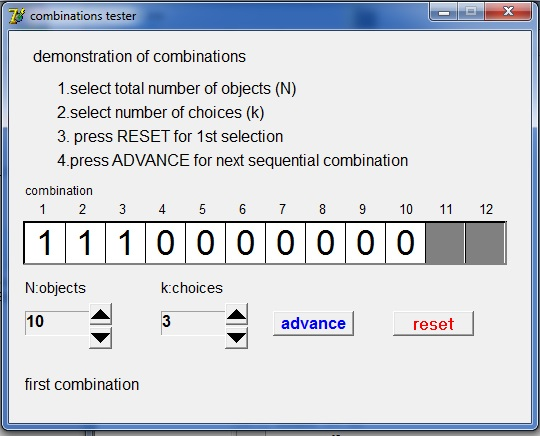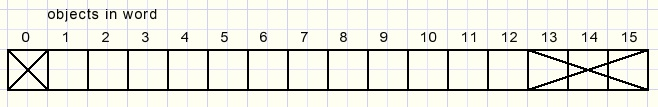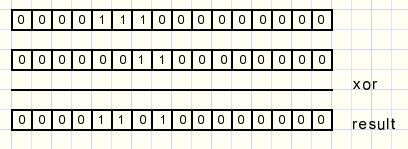A combinations counterdownload exerciser programdownload complete Delphi-7 source code

What is a combination?

Say we have to select 3 people from a group of 10 for corvée.
A selection is called a combination.
The following questions arise:
1.how many different selections are possible?
2.how to find the next selection given the previous one?

A short introduction to refresh our mind.
10 objects may be sorted (placed in line) in 10.9.8.7.6.5.4.3.2.1 = 10! ways.
10! is shorthand, pronounce: ten faculty.
So 4! = 4.3.2.1 = 24.

The eight characters of the word watchdog may be written in 8!= 40320 sequences.
But what about the word paraguay.
The characters "a" are the problem.
So first we make the a's different by painting them in different colors.
Now there are 8! ways to write the characters in a different order.
The three different a's have 3! = 6 different sequences.
So, 8! has 3! times to many sequences and the right answer is 8!/3!= 6720.
Using the characters of "paraguay" we may write 6720 different "words" (most meaningless).

Back to the first problem.
We selected 3 people out of a group of 10.
The selection was done by attaching a sheet of paper to each person with written Y (if choosen) or N (if rejected).
This modifies the question to: how many words may be generated using characters YYYNNNNNNN ?

The answer is 10! / (3! . 7!) = 120.
10! would be the number of sequences with all characters different.
However , the Y's have 3! and the N's have 7! different sequences that count for one in reality.
We have to divide 10! by 3! and also by 7!
The previous calculation we call: "the combinations of 3 out of 10".
Notation: C(10,3) = 120.

In general: if given K choices out of N objects, the number of combinations C is:
C(N,K) = N!/(k!.(N-K)!)

The program

Below we see the program at work:The objects are bit positions (1..10).
A "1" means that the bit was selected, "0" means: bit position not selected.
Of course we can link a bit position to any object we want.
The picture shows the first combination of 3 out of 10, the sequence 1,2,3.

The logical next combination is 1,2,4.
This continues until 1,2,10 and next comes 1,3,4......1,3,5.........etcetera.
Pressing the advance button displays the next combination.

The program code

UP_DOWN counters take care of the choice for the amount of objects (N) and the number of ojects selected (K).
The maximum number of objects is 12.
The variables also are named N and K.

The generated combination is in (16 bit word) variable SH bits 1..12 and is displayed in paintbox1.
(SH from shifter)word SH does not use bits 0 and 13,14,15.

RESET

This procedure generates a row of K "1" bits starting at bit 1: the first combination.
Please notice that the lower bits are pictured at left.
This looks more comfortable, proceding left to right.
```procedure resetSH;
//set SH to first combination of K out of N
var i : byte;
begin
SH := 0;
for i := 1 to K do
begin
end;
end;
```

advanceSH is a function which returns true at the next combination
and false if the last combination failed to advance.

This function is far more difficult then the previous one.
In unit1 we notice these variables and constant:
```const maxN = 12;

var N,K : byte;  //K choices out of N objects
SH  : word;
timercode : byte;
```
The avoid erratic button clicking a timer is attached.
This timer repeats actions as long as a button is pressed down.
The timercode instructs the time-out procedure to take the right action.
Please refer to the source code for more details.
```function advanceSH : boolean;
//SH shift counter increment
//use N,K
var count,pos,i : byte;
Done : boolean;
begin
result := false;
count := 0;
pos := N;
Done := false;
repeat
if SH and mask > 0 then                   //test SH bit
begin
SH := SH xor mask;                      //remove 1
inc(count);                             //count removed 1
if pos + count <= N then                //test space to shift
begin
Done := true;
result := true;
end
else
if count = K then Done := true
else dec(pos);
end
else dec(pos);
until Done;
for i := count downto 1 do                 //add removed bits
begin
end;
end;
```
In advanceSH we notice the variables:
- mask (word): one bit set, used to select a bit in SH
- pos (byte): the positie of the "1" bit in mask
- count (byte): the number of removed "1" bits in SH
Two logical functions are used: and , xor.
For completeness: this is their function:
 A B A and B A xor B 0 0 0 0 0 1 0 1 1 0 0 1 1 1 1 0

Using and we select bits:xor complements bits. A xor with "1" turnes a "1" into a "0" and a "0" into a "1".
Using xor we can set or reset a bit.Below are two examples of the algorithm in action.
1.
(numbers listed in binary, lower bits at left)
First combination 1000 (1 of 4)
Next combination must be 0100.
SH=1000
5. repeat 2,3,4 until pos=1
7. (pos + count) <= N: Yes--> mask shl 1 (next bit); exit repeat loop
8. (count > 0)? Yes-->set mask in SH, repeat 8.
9. return true.
The first "1" bit in SH is searched from right to left.
This bit is reset to "0". Variabele count increments to remember the removed "1" bit.
If pos+count > N there is no space to shift the "1" bit right.
In this case the search is continued for a lower "1" bit.
After exiting the repeat loop, the removed "1" bits are inserted again.

2.
Given combination is 000000000111 (last of 3 out of 12).
SH=000000000111 (binary)
1. count=0;pos=12;
Please refer to the source code for more details.